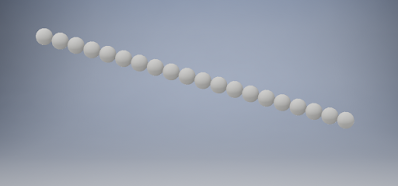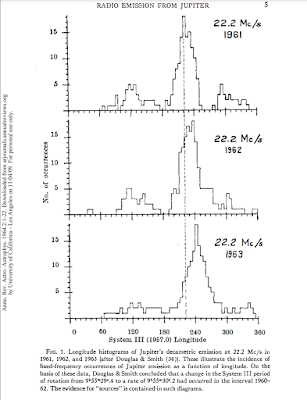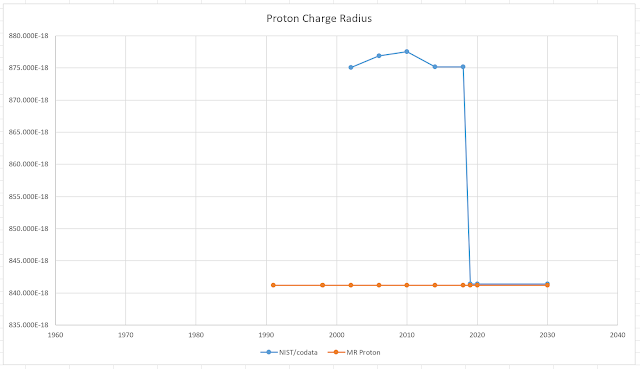## Saturday, May 29, 2021

### Crystalline Lattice Analysis of the Speed of Sound in Metallic Hydrogen (Simple Version - WIP) Sound to Light Sonoluminescencecreditfrom: https://forums.autodesk.com/t5/inventor-forum/3d-array-of-spheres/td-p/8054413

The spacing of the protons, center to center, is $2r_p$. For a standing wave phononic vibration in the crystal, proton to proton spacing is $1\over2$ wavelength, so the shortest standing wave wavelength is $4r_p$, thus the $\pi\over2$? in:https://phxmarker.blogspot.com/2021/05/speed-limit-of-sound-fine-structure.html

$$f_u={v_u\over\lambda_u}$$
$$v_u=c\sqrt{{\pi\over2}r_pR_H}$$
$$\lambda_u=4r_p$$

Wavelength of the sound is $4r_p=3.3656 femtometers$

A subharmonic of a factor 178,274,305 would bring the frequency down to the middle the visible spectrum:Vibrating a crystal of metallic hydrogen at 60GHz would make it radiate in the visible spectrum?
(need to revisit basics - Work In Progress -WIP)
(I would imagine there is a lot of noise in space)

Modifications for Tension-Pressure and density:

$$f_u={v_u\over\lambda_u}\sqrt{T\over\mu}$$
$$v_u=c\sqrt{{\pi\over2}r_pR_H}$$
$$\lambda_u=4r_p$$
$$f_u=c\sqrt{{\pi\over32}{R_H\over r_p}}\sqrt{T\over\mu}$$

Might have to review crystal resonators to progress:

Once the equation is corrected for Pressure/density/elasticity/temperature or whatever, perhaps Jupiter's 22.3MHz emmissions can be simply explained.
Moar coming.saucesource

Some effect like this, however, with the protons+ moving via phonon standing wave in a periodic 3D metallic crystal, for when the phonon frequency equals the photon frequency, however, even that assumption may be wrong... (this is LIVE derived crash and burn, results will verify over time):https://en.wikipedia.org/wiki/Bremsstrahlung

## Saturday, May 8, 2021

### Speed Limit of Sound - Fine Structure Constant and the Proton to Electron Mass Ratio

Speed limit of sound is limited by the speed of light, proton radius, and the Rydberg constant:
(this defines a new experiment / series of experiements to determine the proton radius)https://advances.sciencemag.org/content/6/41/eabc8662*
$$v_u=\alpha({m_e\over{2m_p}})^{1\over2}c$$

using for the proton to electron mass ratio:
$${m_p\over m_e}={\alpha^2\over\pi r_pR_H}$$

$$v_u=c\sqrt{{\pi\over2}r_pR_H}$$

$$r_p={2\over{\pi R_H}}{({v_u\over c})}^2$$

And there you have it, another simple result from good old fashioned work!

It looks like you have a sort of Rydberg Molasses that slows the speed of light down as it traverses the proton radius a quarter wave at a time, thus the ${\pi\over2}$ quarter wave phase-factor, or some rms $\sqrt2\over2$ crystal lattice averaging factor.

* "Our result expands the current understanding of how fundamental constants can impose new bounds on important physical properties."
Other notes:

$$({v_u\over c})^2={\pi\over2}r_pR_H$$
This clearly sheds Light on the geometric reality of the Universe.

™=That's Mine

Assuming the photon and phonon are the same frequency:
$$f_{phonon}=f_u$$
$$f_{photon}={c\over\lambda}$$
$$f_u={v_u\over\lambda_u}$$
$${c\over\lambda}={v_u\over\lambda_u}$$
$${v_u\over c}={\lambda_u\over\lambda}$$
$$({v_u\over c})^2=({\lambda_u\over\lambda})^2$$

$$({\lambda_u\over\lambda})^2={\pi\over2}r_pR_H$$
Moar later to check correlation in k-space/momentum space.https://en.wikipedia.org/wiki/Metallic_hydrogen#Claimed_observation_of_solid_metallic_hydrogen,_2016https://en.wikipedia.org/wiki/Delocalized_electron

The Surfer, OM-IV

## Saturday, March 27, 2021

### Proton Radius: Willfully or Ignorantly a Puzzle?

A simple review of the existing derivations of the foundations of Solid-State theory, and examination of the derivation for the Rydberg constant/equation that puts constraints on the other constants, one can see it appears willful that the terms involving the electron mass and proton mass ratio are dropped.

The reduced mass approximation is an approximation to a 2 "body" problem.  $\mu$, reduced mass,

$$\mu={m_pm_e\over{m_p+m_e}}$$
$$\mu={m_e\over{1+{m_e\over m_p}}}$$
$$\mu=m_e^*$$ reduced mass or effective mass of electron*
$$\therefore m_e^*<m_e$$
Just** turning the terms for the mass ratio into an effective mass ends up dropping the proton radius from the equation,the Rydberg equation, the equation that puts constraints on MANY other key constants.

*effective mass can be determined via experimental or theoretical techniques for periodic arrays of atoms, energy wells, created by crystals (physical atom placement, nanotechnology, EM periodic, or phonon (sound vibration) periodic, HOWEVER, we are talking about a single hydrogen atom for the purpose of solving for some constants of a solution of a characteristic wave equation for a single atom in the aether that is a simultaneous solution simply for determination of the constants BEFORE everyone runs off crazy with ill thought out experiments and missing the boat and ending up with all kinds of puzzles and unsolved physics problems. (I'm talking about the mainstream funded science flow, so yes, that means (You)).  Once the theory is mathematically fully solved, then it's time to compare to experiments where necessary for verification and investigative purposes.

**In other words, do not confuse the solution of one problem with the solution of another, i.e., solid-state periodic array assumptions so one can just treat the electron motion in the anlaysis, very similar to QED, is an approximation to the free atom. Fully solve the single free atom with the constants from the boundary conditions - just like real mathematicians*** do.

***Mathematicians would fully solve the general case for the wave equation and the physicist would point out the boudary conditions which enforce a set of equations that define the specific problem being solved since the general case for a second order fully complex wave function (prior to being reduced to the Schrödinger Wave Equation) is not close form solveable (if the general case actually is then all of what this blog is talking about has already been solved and there is no proton puzzle)

In other news, a double witching or whatever with silver 3/28 & 4/1 with deliveries a month later.  Will they have the silver?

The Surfer has been watching Silver since the 60s...

PS: Reduced mass concept works for technology such as the Solid-State devices we have and the Weyl fermion ideas thrown about, however, approximation techniques have to be CAREFULLY applied and used when doing Fundamental Fysiks!

The Surfer, OM-IV

## Wednesday, March 24, 2021

### Throwing in a Pi ($\pi$) Versus Throwing in the Tau ($\tau$) or "Who Threw Those Pies?" (Never Give Up)

Approximate work here: https://ocw.mit.edu/courses/physics/8-04-quantum-physics-i-spring-2016/video-lectures/part-3/

The Surfer, OM-IV

## Sunday, March 21, 2021

### Explanation of Why Mainstream Analysis & Thinking CANNOT Resolve Measurement Versus Theory for The Proton Radius Puzzle

Here* is why the standard model, QFT, QED, QCD or whatever, cannot calculate a proton radius that agrees with measurement.

It has to do with reductionism and reduced mass. Simply, more precisly and concisely than before, examine the way the terms are added to the ground state energy (from wave equation analysis), for example,  from Pohl's recent video on the proton radius puzzle:

Bohr Quantization

2 particle problem, the proton and electron of the $^1$H atom, reduced to Solid-State (Squalid State) analysis:Simple case: parabolic, isotropic dispersion relationIntermediate case: parabolic, anisotropic dispersion relationAdding the radius term and other (measurement analysis is correct, however, the theory has to wholly solve to give a prediction such as this blog and Haramein's work which correctly predicts the proton radius)

These are parts the terms, formulations,  and coefficients of the Schrödinger Wave Equation where as the Full Wave Equation for a single hydrogen atom (2 particle problem, closed form analytical solution for a single hydrogen atom exists and is the only atom with a complete analytical solution) may be  needed to be used for a more precise mathematical treatment to derive the proton radius (as already done by this blog and by the author circa 1991).

Note how the terms are ratios of Energy to Mass AND, a starting point is the Bohr model, and since the Bohr model is incorrect, that's where the Standard Model comes in and can add more terms for newly discovered effects.

So, from the Bohr model, more and more terms are added.

Note, again, however, the inverse relationship wrt to MASS being in the denominator. Since the effective mass / reduced mass of the electron is slightly less than the actual rest mass of the electron, 1 over the effective mass term will always be greater than the 1 over electron rest mass term.  No matter how many terms one adds (assuming positive mass, positive energy as negative may not be reality) the effective mass will always diverge from the actual electron mass, thus any other derivations, like for the proton radius, will diverge from measurements. A full analysis must be done to extract the proton radius as well as the other constant in the Rydberg equation.  In other words, do not drop the ${{m_e}\over{m_p}}$ term simply because it is less than experimental error and allows a closed form solution.  Keep the term and do a numerical solution via iteration - Numerical Methods solve precisely.

$${1\over{m^*_e}} + {1\over{N}}>{1\over{m_e}}$$
where
$m_e=$ mass of electron
${m^*_e}={{m_pm_e}\over{m_p+m_e}}=$ reduced mass (effective mass)
$N=$ New terms from "more accurate standard model"

This can easily be seen by realizing:
$$m^*_e<m_e$$
$${1\over{m^*_e}}>{1\over{m_e}}$$

Therefore, the way to solve the problem is to carefully consider the 2-body problem before reducing it via reductionism into a 1-body problem.  I.e., more carefully derive the Rydberg equation from the Full Wave Equation.  All in the framework of 0°K absolute zero, no phonons, since we are establishing a reference, the proton, the instrument with which the characteristics of the single hydrogen atom and its vacuum environmental dynamic can be predicted & extracted.

This is the best example of an experimental approach (measurement & technology) dominating over an actual well thought out application of the theory and thus clouding the discussion and results.

And it has been cloudy since that proton work in Stanford in 1968 and before...

4/2/2021 update -MR Proton, aka, The Surfer:
A recent attempt, using QCD, at the proton radius:

*I've been doing this kind of analysis since 1984 - 1989 where I wrote a process manual or two  at Texas Instruments headquarters for an advanced Integrated Circuit (IC) analog process. Some of my calculations or thoughts on the proton to electron mass ratio may be in my engineering notebooks in the TI library in South Building(?) Dallas, Tx.

## Wednesday, December 23, 2020

### Proton Charge Radius vs YearProton Charge Radius vs. Year (NIST/codata & MR Proton*)(perhaps I should send NIST a congrats and welcome aboard letter!)

MR Proton doesn't flinch, plays chicken almost as good as POTUS45!

Some of Pohl's work:

And another important measurement/constant, the Rydberg constant:the most accurately determined fundamental constant AND it's wrong R O N G wrong**
(it's only good to about 1930 numbers 2-4 decimal places)
(will be defined by this work)

* this work
** corrected by this work/blog/solution to proton radius
The Surfer, OM-IV

## Friday, December 18, 2020

### STANDARD DIGITAL SOLDIER PROCEDURES

STANDARD DIGITAL SOLDIER PROCEDURES

Get Everyone at ONCE and HIT 'Them' With Everything You Got! THINK PICARD MANUEVER! [POST STORM - THE WAVE]

 MEME MEME and MEME some MOAR! Stay Focused on Current #HOTTOPICS
 Pick 2-3 hashes from the target area https://www.trendolizer.com Hijack them to get your #hash out.
 Post @ 10-11Am | 12-3Pm | 6-9Pm EST
 Always Post meme or Cap
 Use .@them to respond to all their following.
 Remember to be Quirky in your posts.
 RT other's tweets and Report Bots
 ReTweet LASERFAST. Use https://tweetdeck.com
 Information Warfare https://archive.is/E0oJm

#TRUMP2020 #MAGA #WWG1WGA #FGNC #ANONYMOUS

Social Media Warfare Armory
>>>/warroom/925         ————————————–——– More Twitter Tools and Services
Learn Digital Warfare
>>>/warroom/5684        ————————————–——– Lessons in Digital Warfare #1
>>>/warroom/5700  AN OFF BREAD COLLECTION OF VOTER ELECTORAL FRAUD

TOOLS
* Deleted Trump Tweets: https://factba.se/topic/deleted-tweet
* Notables Aggregator: https://wearethene.ws
* Video Pastebin https://videobin.org/
https://timetravel.mementoweb.org/
https://archive.today
https://archive.org
https://anonfile.com
https://postimg.cc
https://www.trendolizer.com/
https://www.trendsmap.com/
gimp - raster | inkscape - vector >>8723
https://gimp.org graphics editor
All My Tweets: Archive/Scan any Twatter account in text form: https://www.allmytweets.net/
Searchable Commercial Aviation Incident List: https://avherald.com
* gimp - raster | inkscape - vector >>8723
* https://gimp.org graphics editor
* 1160-4149-8629-5271-8713-0586 key adobe lightroom 5.3
* All My Tweets: Archive/Scan any Twatter account in text form: https://www.allmytweets.net/
* Searchable Commercial Aviation Incident List: https://avherald.com
* Searchable Hussein WH visitor list: https://qest.us/obamavisitors
* Qcode Guide to Abbreviations: https://pastebin.com/UhK5tkgb
* Q Happenings Calendar 2018: https://mega.nz/#F!KPQiBJiY!dK3XRe4RYoXgWq_85u4-yg
* Legal News: https://www.justice.gov/usao/pressreleases
* Get your Q clocks anytime (0 - 59 min past posts): https://q-clock.com
* New google doc tracking deplatforming/bans on social media: >>6484178
* Bash Script - https://pastebin.com/JbRNzN3J
* Spreadsheet and Resources - https://pastebin.com/EygV3gRC
* Q Cards: https://genesis55.github.io/QCards/
* Search political donations: https://www.opensecrets.org/donor-lookup/
* Collection of declassified gov docs: https://www.theblackvault.com/documentarchive
* MKU project documents: https://www.illuminatirex.com/list-of-mkultra-subprojects/
* List of illuminati symbols: https://illuminatisymbols.info/
* List of some alt news sites: https://start.io/qanon/alternativenewsblogs
* https://timetravel.mementoweb.org/ (we dont use http around here)
* Stock Movement Scraper: https://qest.us (for seeing LARGE movements of \$)
* Federal Procurement Data System: https://www.fpds.gov/fpdsng_cms/index.php/en/
* Federal Judicial Court dataset from 93 Federal Districts - Searchable db: https://bad-boys.us/

## Saturday, December 12, 2020

### Probability - Oneness - Wholeness

The Power of One
This guy has no clue!

When discussing probability and odds and such, mathematically, the concept of 1 comes up.  The probability of an event is between 0 and 1.

0: it didn't happen (Shaggy)
1: it surely did happen (Shaggy's pregnant girlfriend)

So, if yer doing odd experiments about odds and such, all the little pieces of the puzzle yer working on have to be between 0 and 1 and the sum of them ALL is 1.

If yer missing anything in your analysis of the "whatever" the sum of all odds won't quite add up to one, and if it adds up to greater than one, then you got something wrong - R O N G - wrong.

Likewise, when you're adding up energy in physics.  If you have a theory that keeps track of ALL the energy and sums it up, then compares that sum to the measured sums of experimental energy, IF there is ANY missing energy, that is of GREAT interest to scientists.

Any excess energy or missing energy is of GREAT interest.

Thus are the great physics/science problems of today, just sitting there, Grasshopper, like a pebble to be snatched from the Master's Hand.

The mainstream's Rydberg equation doesn't quite add up to 1.

Good day!

The Surfer, OM-IV

# Unprecedented accuracy in quantum electrodynamics: Giant leap toward solving proton charge radius puzzle

I'd like to see their work on what their theory predicts or expects for the proton radius.  So far what they offer does not fit, not matter how many terms are added their polynomial does not fit (research series of polynomial no fit papers) the gap between their theory and the measured.

The Surfer, OM-IV

## Thursday, November 26, 2020

### Three (3) Parts of the Physics Required to EVEN Begin to Understand the Interstellar Context

Dan really gets to the point and summarizes Keystone points of information required to EVEN begin to talk about interstellar reality (the way Nature truly is and behaves).

Interstellar Value of Humans: Bliss-Radiance Fractal Wireless Energy-Pyramids and Stargates

Galactic History series
1) Ascension physics and more https://youtu.be/h_E_PHa9nhY
2) Interstellar Value of Humans: https://youtu.be/Dp6BQxjNfd4
3) Star History and Value of Humans: https://youtu.be/bnFs5pEbLAU
4) Physics of God & ET Origins of Religion: https://youtu.be/1rwLiRJgQVM

The Surfer, OM-IV

## Tuesday, October 20, 2020

### Excel Spreadsheet with VBA Macro for ROOTS #2, Initial Error

A good starting point would be to examine the coefficients (constants) of the equation for the Rydberg Constant and comment on which ones are in minor error, major error, and why.

Feynman gave up on the question why and ridiculed philosophers.  I don't think he quite realized the box he had trapped himself in so deeply.  Why always points to more and possible purpose.  Richard dug through the cargo like no other cult scientist.  Anyway, I digress.......

The glaring error is the -4% on the proton radius.  Mainstream (NIST/CODATA) doesn't have a correlation of the proton radius with the proton mass nor any of the other physics constants.  While the Rydberg constant equation puts constraints on other constants (due to correlation via Rydberg equation of the standard model) but mainstream doesn't include the proton radius, thus missing the boat.

Tying in the proton radius to the full Rydberg equation not only then puts constraints on the proton radius like it does the other constants, it also becomes an equation that can be solved via iteration.

(paste error table here an initial CODATA starting values)...

(Work In Progress)